The Japanese Bonsai specialist
Direct order Contact Help / Services NewsletterManeki-neko white lucky charm cat

Maneki-neko 招き猫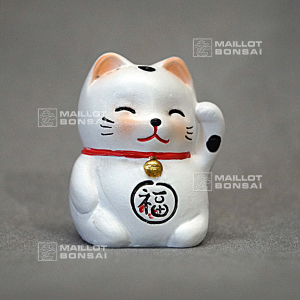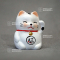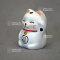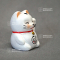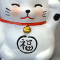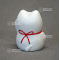ref. : 10460

2,20

Available quantity : 16Order

Description

maneki-neko招き猫 often named the lucky charm cat.

Hand painted in white with an ideogram graved on the belly, some red touches on the ears and a small bell on the neck.

Japanese traditional statue in terracotta that represents a cat, sitting down and raising up the paw to the ear. Traditional object, often presented in the windows displays of shops, near the cash registers in commercial centers, in pachinko (japanese casino) rooms etc...

This little cat is supposed to attract wealth.

Maneki 招き comes from the verb maneku 招く that means "to invite" in japanese (meaning to summon or greet) and neko 猫 means "cat". Then it is literally "the cat that invites". The left paw is supposed to attract customers and the right money.

Maneki-neko can be found in houses, as a money box, key rings or other objects.

#maneki 5.6 #neko 5.4 #japanese 3.8 #white 3.5 #charm 3.5 #lucky 3.5 #that 3.4 #traditional 3.1 #figurines 2.9 #supposed 2.8

Formule
(( ROUND((CHAR_LENGTH(b.article_nom)-CHAR_LENGTH(REPLACE(b.article_nom, 'maneki', '')))/LENGTH('maneki')) + ROUND((CHAR_LENGTH(b.article_description)-CHAR_LENGTH(REPLACE(b.article_description, 'maneki', '')))/LENGTH('maneki')) ) * 5.6) + (( ROUND((CHAR_LENGTH(b.article_nom)-CHAR_LENGTH(REPLACE(b.article_nom, 'neko', '')))/LENGTH('neko')) + ROUND((CHAR_LENGTH(b.article_description)-CHAR_LENGTH(REPLACE(b.article_description, 'neko', '')))/LENGTH('neko')) ) * 5.4) + (( ROUND((CHAR_LENGTH(b.article_nom)-CHAR_LENGTH(REPLACE(b.article_nom, 'japanese', '')))/LENGTH('japanese')) + ROUND((CHAR_LENGTH(b.article_description)-CHAR_LENGTH(REPLACE(b.article_description, 'japanese', '')))/LENGTH('japanese')) ) * 3.8) + (( ROUND((CHAR_LENGTH(b.article_nom)-CHAR_LENGTH(REPLACE(b.article_nom, 'white', '')))/LENGTH('white')) + ROUND((CHAR_LENGTH(b.article_description)-CHAR_LENGTH(REPLACE(b.article_description, 'white', '')))/LENGTH('white')) ) * 3.5) + (( ROUND((CHAR_LENGTH(b.article_nom)-CHAR_LENGTH(REPLACE(b.article_nom, 'lucky', '')))/LENGTH('lucky')) + ROUND((CHAR_LENGTH(b.article_description)-CHAR_LENGTH(REPLACE(b.article_description, 'lucky', '')))/LENGTH('lucky')) ) * 3.5) + (( ROUND((CHAR_LENGTH(b.article_nom)-CHAR_LENGTH(REPLACE(b.article_nom, 'charm', '')))/LENGTH('charm')) + ROUND((CHAR_LENGTH(b.article_description)-CHAR_LENGTH(REPLACE(b.article_description, 'charm', '')))/LENGTH('charm')) ) * 3.5) + (( ROUND((CHAR_LENGTH(b.article_nom)-CHAR_LENGTH(REPLACE(b.article_nom, 'that', '')))/LENGTH('that')) + ROUND((CHAR_LENGTH(b.article_description)-CHAR_LENGTH(REPLACE(b.article_description, 'that', '')))/LENGTH('that')) ) * 3.4) + (( ROUND((CHAR_LENGTH(b.article_nom)-CHAR_LENGTH(REPLACE(b.article_nom, 'traditional', '')))/LENGTH('traditional')) + ROUND((CHAR_LENGTH(b.article_description)-CHAR_LENGTH(REPLACE(b.article_description, 'traditional', '')))/LENGTH('traditional')) ) * 3.1) + (( ROUND((CHAR_LENGTH(b.article_nom)-CHAR_LENGTH(REPLACE(b.article_nom, 'supposed', '')))/LENGTH('supposed')) + ROUND((CHAR_LENGTH(b.article_description)-CHAR_LENGTH(REPLACE(b.article_description, 'supposed', '')))/LENGTH('supposed')) ) * 2.8) + (( ROUND((CHAR_LENGTH(b.article_nom)-CHAR_LENGTH(REPLACE(b.article_nom, 'attract', '')))/LENGTH('attract')) + ROUND((CHAR_LENGTH(b.article_description)-CHAR_LENGTH(REPLACE(b.article_description, 'attract', '')))/LENGTH('attract')) ) * 2.7)

Secure paymentDelivery

Our logistic partners :04 74 55 23 48
Pépinière MAILLOT-BONSAÏ
Le Bois Frazy
01990 RELEVANT - FRANCE
on appointment